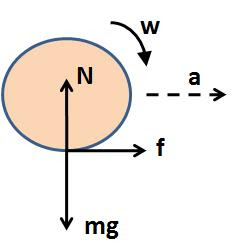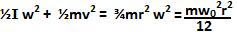Thursday, December 18, 2008

Irodov Problem 1.262As soon as the spinning cylinder (say in the clockwise direction) is placed on the surface it initially slips (the point of contact of the cylinder with the surface - the lowest point of the cylinder, is moving backwards at a speed w0r relative to the surface). The force of friction f acts on it to resist this slipping between the lowest point of the cylinder (the point of contact of the cylinder with the surface) and the surface and as a result accelerates the cylinder forward. The friction force f also generates a retarding torque that opposes the spinning motion and reduces the cylinder's angular velocity. If the velocity of the cylinder in the forward direction at any point of time is v and its angular velocity due to spinning motion is w then the velocity of the lowest point of the cylinder is wr - v backwards. As v increases (due to the force f) and w decreases (due to the resitive torque fr) at some point of time when wr-v = 0, the net velocity of the lowest point of the cylinder becomes 0. At this point there is no relative motion between the surface and the point of contact of the cylinder and the cylinder begins to roll and the force of friction ceases to act.

The only force acting on the mass in the horizontal direction is f and if the acceleration of the cylinder is a then,Suppose that the angular acceleration of the cylinder is b, then we have,There are two forces acting acting in the vertical direction, i) the normal reaction N from the surface and ii) the force of gravity mg acting downwards. There is no acceleration for the cylinder in the vertical direction and so we have,The maximum friction force that can be offered is Nk and so this is the friction force f that is generated on the cylinder. So using (3) we have,From (1) and (4) we have,From (5) and (6) we have,The friction force is the only force responsible for reducing the energy of the cylinder. Thus the difference between final and initial kinetic energies is the work done by the firction. Initially the cylinder has no translational motion, it only spins and its kinetic energy is purely due to rotational motion given by. Finally however, the cylinder has both translational and rotational motions and thus the total kinetic energy is the sum of both these parts given by. The work done by friction is thus given by,